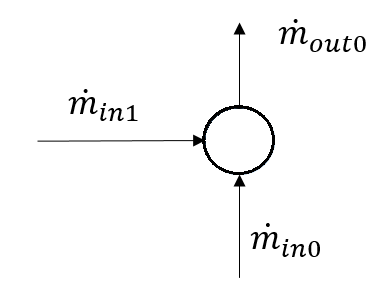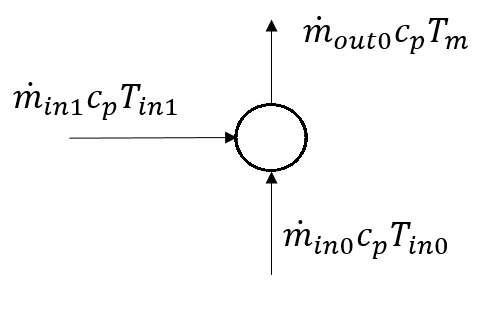# Junction

## Create Function

For creating a single junction:

create_junction(net, pn_bar, tfluid_k, height_m=0, name=None, index=None, in_service=True, type='junction', geodata=None, **kwargs)

Adds one junction in table net[“junction”]. Junctions are the nodes of the network that all other elements connect to.

Parameters
• net (pandapipesNet) – The pandapipes network in which the element is created

• pn_bar (float) – The nominal pressure in [bar]. Used as an initial value for pressure calculation.

• tfluid_k (float) – The fluid temperature in [K]. Used as parameter for gas calculations and as initial value for temperature calculations.

• height_m (float, default 0) – Height of node above sea level in [m]

• name (string, default None) – The name for this junction

• index (int, default None) – Force a specified ID if it is available. If None, the index one higher than the highest already existing index is selected.

• in_service (boolean, default True) – True if the junction is in service or False if it is out of service

• type (string, default "junction") – not used yet - Designed for type differentiation on pandas lookups (e.g. household connection vs. crossing)

• geodata ((x,y)-tuple, default None) – Coordinates used for plotting

• kwargs – Additional keyword arguments will be added as further columns to the net[“junction”] table

Returns

index - The unique ID of the created element

Return type

int

Example
>>> create_junction(net, pn_bar=5, tfluid_k=320)


For creating multiple junctions at once:

create_junctions(net, nr_junctions, pn_bar, tfluid_k, height_m=0, name=None, index=None, in_service=True, type='junction', geodata=None, **kwargs)

Convenience function for creating many junctions at once. Parameter ‘nr_junctions’ specifies the number of junctions created. Other parameters may be either arrays of length ‘nr_junctions’ or single values.

Parameters
• net (pandapipesNet) – The pandapipes network in which the element is created

• nr_junctions (int) – Number of junctions to be created.

• pn_bar (Iterable or float) – The nominal pressure in [bar]. Used as an initial value for pressure calculation.

• tfluid_k (Iterable or float) – The fluid temperature in [K]. Used as parameter for gas calculations and as initial value for temperature calculations.

• height_m (Iterable or float, default 0) – Heights of nodes above sea level in [m]

• name (Iterable or string, default None) – The names for these junctions

• index (Iterable(int), default None) – Force specified IDs if they are available. If None, the index one higher than the highest already existing index is selected and counted onwards.

• in_service (Iterable or boolean, default True) – True if the junctions are in service or False if they are out of service

• type (Iterable or string, default "junction") – not used yet - Designed for type differentiation on pandas lookups (e.g. household connection vs. crossing)

• geodata (Iterable of (x,y)-tuples, default None) – Coordinates used for plotting

• kwargs – Additional keyword arguments will be added as further columns to the net[“junction”] table

Returns

index - The unique IDs of the created elements

Return type

array(int)

Example
>>> create_junctions(net, 200, pn_bar=5, tfluid_k=320,
>>>                  height_m=np.arange(200))


## Component Table Data

net.junction

 Parameter Datatype Value Range Explanation name string Name of the junction pn_bar float $$>$$ 0 Rated pressure of the junction [bar]. Also the initial value for the calculation. tfluid_k float $$\ge$$ 0 Fluid temperature at junction [K] height_m float $$\ge$$ 0 The height above sea level of the junction [m] in_service boolean True / False Specifies if the bus is in service. type string p or t or pt Type variable to classify junctions

net.junction_geodata

 Parameter Datatype Explanation x float x coordinate of bus location y float y coordinate of bus location

## Physical Model

The equations added by a junction correspond to a mass flow balance for hydraulic calculations.\begin{align*} \sum_{n=0}^N \dot{m}_{in_n} - \sum_{l=0}^M \dot{m}_{out_l} = 0 \end{align*}

For temperature calculations, the thermal power carried by the incoming fluids is balanced:\begin{align*} \sum_{n=0}^N \dot{m}_{in_n}c_p T_{in_n} - \sum_{l=0}^M \dot{m}_{out_l} c_p T_{m} = 0 \end{align*}

## Result Table Data

net.res_junction

 Parameter Datatype Explanation p_bar float The junction pressure [bar] t_k float The junction temperature [K]